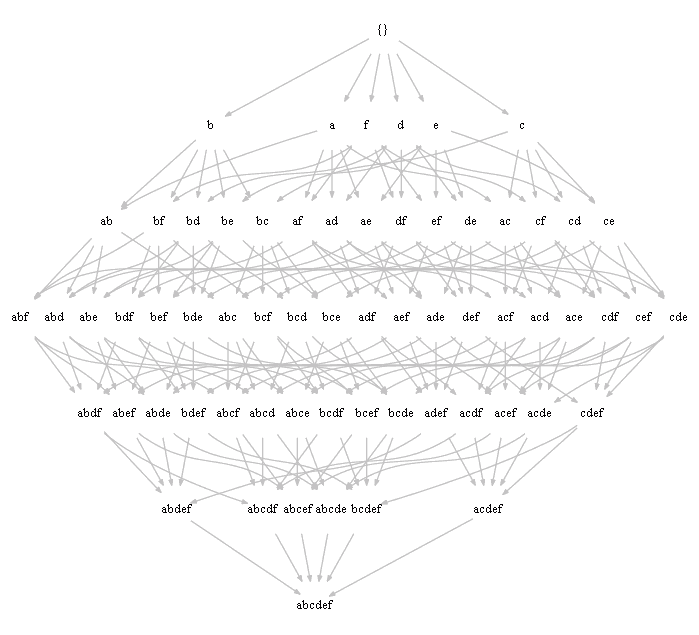ML Wiki

Local Patterns Discovery

Local Patterns

Suppose that we have the following table:

Wings Beak Webfoot Fly Swim
Owl
Parrot
Flamingo
Penguin

What we can learn from this data?

• Global (mainstream) Patterns/Rules
• All birds have wings and beaks
• Almost all birds fly
• Local Patterns/Rules
• only 25% can swim and fly
• 100% of swimming birds are webfooted

Can reformulate these rules with probabilities

• $P(\text{swim} \land \text{fly}) = 0.25$: 25% of birds can swim and fly
• $P(\text{swim} \ | \ \text{webfoot}) = 1$: 100% of web-footed birds can swim
• $P(\text{webfoot} \ | \ \text{swim}) = 1$: 100% of swimming birds are web-footed ones

Confidence and Support

Notation

• items: set of distinct literals: $\{ a, b, c, ...\}$
• itemsets: any combination of items $\{ a, f, ... \}$
• language: all possible itemsets for the set of items
• dataset: a multiset (i.e. a set that allows duplicates) of itemsets
• an itemset is frequent if it happens more than some certain threshold

Search space - Lattice

• all possible combinations of our items
• with arrows showing inclusions of one itemset into another
•Support

Support of itemset $X$

• proportion of transactions in dataset $D$ that contain $X$
• $\text{supp}(X, D) = \cfrac{ \big| \{ T \in D \ | \ X \subseteq T \} \big| }{ | D | }$
• or simpler, $\text{supp}(X, D) = \cfrac{ \text{freq}(X, D) }{ | D | }$

Support of an association rule $X \to Y$

• $\text{supp}(X \to Y, D) = \text{supp}(X \cup Y, D)$

Confidence

Confidence of an association rule $X \to Y$

• $\text{conf}(X \to Y, D) = \cfrac{\text{supp}(X \to Y, D)}{\text{supp}(X, D)} = \cfrac{\text{supp}(X \cup Y, D)}{\text{supp}(X, D)}$

Example

Consider this

• items: ${A, B, C, D, E, F}$
• $\left[ \begin{matrix} {\color{red}{1}} & {\color{red}{1}} & {\color{red}{1}} & 0 & 0 & 0 \\ 1 & 0 & 1 & 1 & 1 & 1 \\ {\color{red}{1}} & {\color{red}{1}} & {\color{red}{1}} & 0 & 0 & 0 \\ 0 & 0 & 0 & 1 & 1 & 0 \\ \end{matrix} \right]$

• $\text{supp}(AC) = 3/4$
• in 3 cases out of 4 the statement is true
• $\text{supp}(AC \to B) = \text{supp}(ABC) = 2/4$
• this rule is true in 2 cases out of 4
• $\text{conf}(AC \to B) \cfrac{\text{supp}(ABC)}{\text{supp}(AC)} = \cfrac{2/4}{3/4} = \cfrac{2}{3}$
• intuition: $AC$ is true in 3 cases, and out of these 3, $ABC$ is true only in 2

Example 2

${A, B, C, D, E, F}$

• $T_1 = \{A,B,D,E\}$
• $T_2 = \{A,B,C,D,F\}$
• $T_3 = \{B,D,F\}$
• $T_4 = \{C,E,F\}$

Find itemsets with support at least 50%

• $\text{supp}(X)$ - proportion of transactions that contain $X$
• here we want to find rules $X \to A$ with support 50% or more

Let's calculate it for a couple of rules

• $\text{conf}(B \to A, D) = \cfrac{2/4}{3/4} = \cfrac{2}{3}$
• $\text{conf}(D \to A, D) = \cfrac{2/4}{3/4} = \cfrac{2}{3}$
• $\text{conf}(BD \to A, D) = \cfrac{2/4}{3/4} = \cfrac{2}{3}$
• $B \to A$ and $D \to A$ are shorter - those rules are usually better

Measures of Interestingness

There could be other measures of interestingness of $X$ in $D_i \subset D$

• Lift: $\text{lift}(X,D_i,D)=\cfrac{\text{supp}(X,D_i)}{\text{supp}(X,D)}$
• Growth rate: $\text{gr}(X,D_i,D)=\cfrac{\text{supp}(X,D_i)}{\text{supp}(X, D - D_i)}$
• how much more support $X$ has in $D_i$ than in $D - D_i$

Problems

Frequent Patterns

• finding patterns with probabilities that exceed a certain threshold
• Descriptive patterns: just combinations of items

e.g.

• $\text{wings}$
• $\text{beak}$
• $\text{flying}$
• $\text{wings} \land \text{beak}$
• $\text{...}$
• $\text{wings} \land \text{beak} \land \text{fly}$

Or, formally

• find $\{ X \ : \ \text{supp}(X, D) \geqslant \text{min_supp} \}$

Association Rules

• Finding all the rules $X \to Y$ such that
• $P(X \land Y) \geqslant \text{min_supp}$ and
• $P(Y \ | \ X) \geqslant \text{min_con}$
• these are predictive patterns

Or, formally

• find $\{ X \to Y \ : \ \text{supp}(X \to Y, D) \geqslant \text{min_supp} \land \text{conf}(X \to Y, D) \geqslant \text{min_conf} \}$

With frequent patterns there are some problems:

• they are frequent, i.e happen a lot of the time
• bus we sometimes want to find some exceptional patterns that occur less rarely

So there are other mining tasks

• Constraint-Based Pattern Mining
• Exception mining
• finding rare features
• Contrast mining
• characterizing the difference between two classes
• Utility-pattern mining
• the frequency is not the only end-user interest
• (e.g., price of items in a supermarket)

Contrast Mining

Mining patterns that compares two or more datasets

Method 1

(with Frequent Pattern Mining)
• Mine all the non frequent patterns in class 1
• Mine all the frequent patterns in class 2
• Intersect results

Method 2

(without frequent pattern mining)
• Mine all the patterns with frequency in class 2 greater than that in class 1